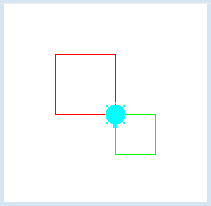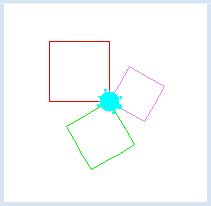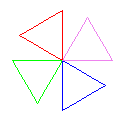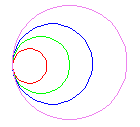deutsch     english    français     Print

## 2.5 PARAMETERS### INTRODUCTION

 When using the command forward() you tell the turtle how far you want it to go, putting the value in the parentheses. Therefore, the value in the parentheses indicates how far the turtle will go forward. It specifies details of the command and is called a parameter: In this case, it is a number that can vary every time that you use forward(). In the previous chapter you defined your own command square(), but in contrast to forward(), the side length of the square is always 100 pixels. In many different cases it would be quite convenient to be able to adjust the side length of the square. How would you do that? PROGRAMMING CONCEPTS: Parameters, passing parameters

### COMMANDS WITH PARAMETERS

 In this program we are defining a square too. Instead of using empty parentheses in the definition of the function square(), we add the parameter name sidelength and use it when calling forward(sidelength). You can thus use the function square many times, each time with a number for sidelength. With square(80) the turtle will draw a square with a side length of 80 pixels, whereas with square(50) it will draw one with a side length of 50 pixels.```from gturtle import *

def square(sidelength):
repeat 4:
forward(sidelength)
left(90)

makeTurtle()
setPenColor("red")
square(80)
left(180)
setPenColor("green")
square(50)
```
Highlight program code (Ctrl+C copy, Ctrl+V paste)

### MEMO

 Parameters are placeholders for values, which can be different each time. When defining a command, you put parameters inside a pair of parentheses after the command name. ```def commandname(parameter): Instructions that use parameter ``` The parameter name can be chosen freely, but it should reflect its meaning. When using the command you put the value of the parameter in parentheses, too. ```commandname(123) ``` Here the parameter value will be 123 for the entire command.

### MULTIPLE PARAMETERS

 Commands can have multiple parameters. With a square you can use def square(sidelength, color) to add parameters for both side length and color. You can then use square much more flexibly. With square(100, "red") the turtle draws a red square with a side length of 100 pixels, and with square(80, "green") it draws a green square with a side length of 80 pixels.```from gturtle import *

def square(sidelength, color):
setPenColor(color)
repeat 4:
forward(sidelength)
left(90)

makeTurtle()
square(100, "red")
left(120)
square(80, "green")
left(120)
square(60, "violet")
```
Highlight program code (Ctrl+C copy, Ctrl+V paste)

### MEMO

 Commands can have multiple parameters. The parameters are written in the parentheses and  separated by commas. ```def commandname(parameter1, parameter2, ...): Instructions that use parameter1 and parameter2 ``` The order of the parameters in the parentheses in the definition of the command must match the order of the values in calling the command.

### EXERCISES

1.

 Define a command triangle(color) with which the turtle can draw colored triangles. Draw 4 triangles with the colors red, green, blue and violet.2.

 Define a command colorCircle(radius, color) with which the turtle draws a colored circle. You can use the command rightArc(radius, angle). Make the adjacent figure.3.

Unfortunately, the following program draws 3 equally sized pentagons instead of the expected differently sized ones. Why is this? Fix it.

```from gturtle import *

def pentagon(sidelength, color):
setPenColor(color)
repeat 5:
forward(90)
left(72)

makeTurtle()
pentagon(100, "red")
left(120)
pentagon(80, "green")
left(120)
pentagon(60, "violet")
```
Highlight program code (Ctrl+C copy, Ctrl+V paste)

4.

Using the command segment(s, w), you tell the turtle that it should move a certain distance s forward and to rotate by a certain angle w:
```def segment(s, w):
forward(s)
right(w)
```
Write a program that runs this command 92 times with s = 300 and w = 151. Using setPos(x, y) you can start with the turtle at a suitable position in the window.

5*.

The turtle will execute two, three, or four-segment movements. Check out the nice graphics in the following cases:

 Number of Segments Values Number of Repetitions 2 forward(77) right(140.86) forward(310) right(112) 37 3 forward(15.4) right(140.86) forward(62) right(112) forwad(57.2) right(130) 46 4 forward(31) right(141) forward(112) right(87.19) forward(115.2) right(130) forward(186) right(121.43) 68